## Laplace's Equation--Toroidal Coordinates

In Toroidal Coordinates, attempt Separation of Variables to Laplace's Equation by plugging in the trial solution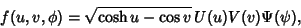(1)

then divide the result by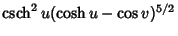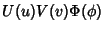to obtain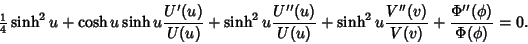(2)

The function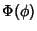then separates with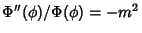, giving solution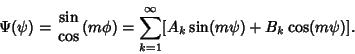(3)

Plugging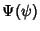back in and dividing by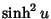gives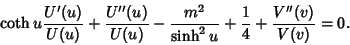(4)

The function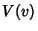then separates with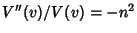, giving solution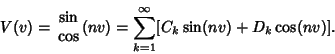(5)

Pluggingback in and multiplying bygives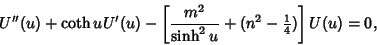(6)

which can also be written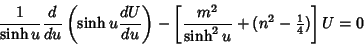(7)

(Arfken 1970, pp. 114-115). Laplace's Equation is partially separable, although the Helmholtz Differential Equation is not.

Laplace's Equation is partially separable, although the Helmholtz Differential Equation is not.

References

Arfken, G. Toroidal Coordinates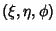.'' §2.13 in Mathematical Methods for Physicists, 2nd ed. Orlando, FL: Academic Press, pp. 112-114, 1970.

Byerly, W. E. An Elementary Treatise on Fourier's Series, and Spherical, Cylindrical, and Ellipsoidal Harmonics, with Applications to Problems in Mathematical Physics. New York: Dover, p. 264, 1959.

Morse, P. M. and Feshbach, H. Methods of Theoretical Physics, Part I. New York: McGraw-Hill, p. 666, 1953.